×
Community Blog Image Recognition Using Edge Detection

Image Recognition Using Edge Detection

Image recognition is a popular technology that can detect, understand, and distinguish images from one another.Image recognition is a popular technology that can detect, understand, and distinguish images from one another. Technologies such as text recognition and facial recognition are all specific applications of image recognition.How is it done?

Understanding the way we perceive objects and images has always been a hot topic for research. Researchers globally have observed that the human eye is very sensitive to the edges of an object. Typically, a person identifies an object by first determining the outline of the object and then processing this information in the visual cortex. Computer scientists have designed sophisticated image recognition systems by emulating the way we recognize images.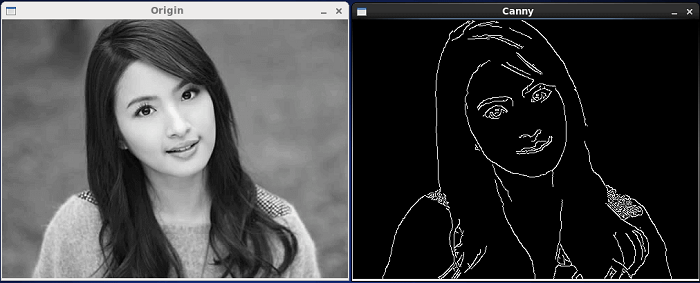The example below is based on a paper by Adit Deshpande, a student at The University of California, titled A Beginner's Guide To Understanding Convolutional Neural Networks. In this paper, he introduces a simple algorithm as the basis of image recognition.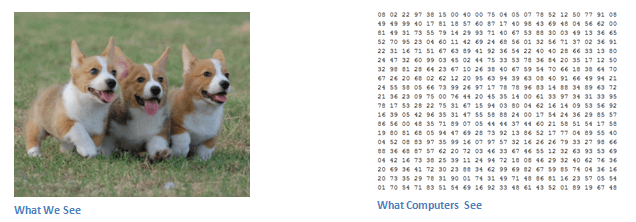Unlike the human eye, a computer can only recognize images as numbers. The above image helps us understand the differences in image perception by a human and a computer. A computer will then perform "image recognition" techniques by determining a pattern from this large matrix of numbers.

Generally, in edge detection, we can convert the color information of every pixel into its grayscale value. To minimize interference, we can downsize the image (such as downsizing the image to 49x49 pixels), thus resulting in a 49x49 matrix.

We can then analyze the matrix section-by-section starting from the top left corner.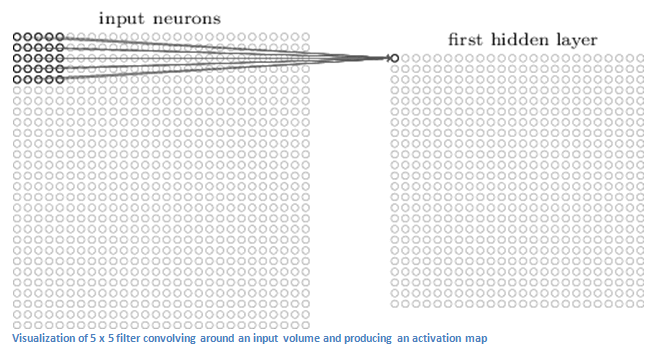Next, we take some existing edge models, such as verticals, right angles, circles, acute angles and so on. The figure below shows a 7x7 matrix of an edge model and its corresponding visualized curve.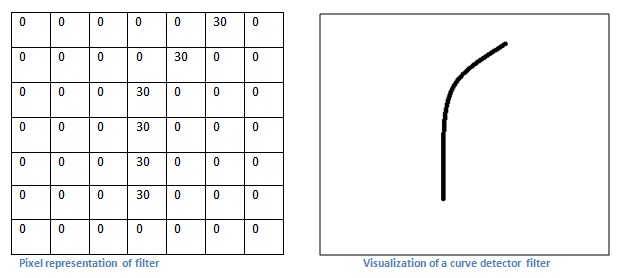Observe how the value of the matrix is nonzero whenever the pixels overlap with the rounded curve.

Now that we have determined an edge filter, let us take it a step higher. The figure below shows a grayscale image of a mouse.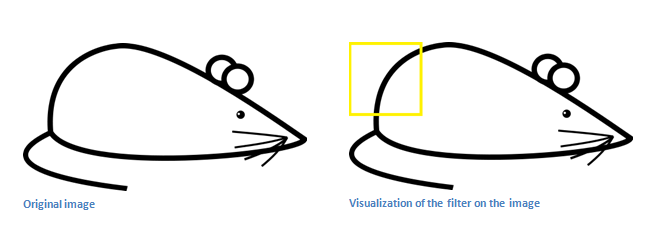Take a section of the image starting from the upper-left corner and obtain its pixel representation. We can then convolve this matrix with the edge filter matrix.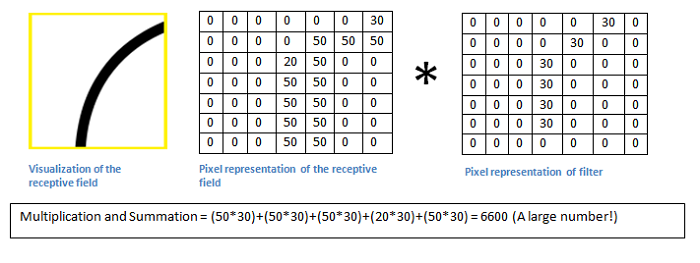The result is 6,600. What does this value indicate? Let's analyze a different section. Move the sampling matrix towards the head of the mouse and perform the same calculations.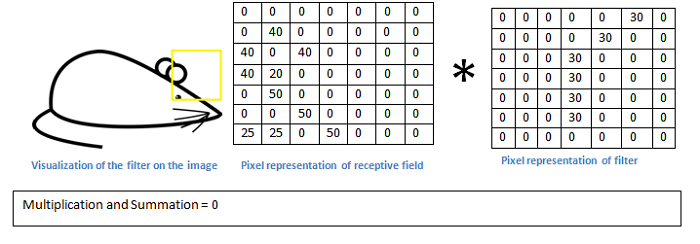Convolving the two matrices produces a value of 0.

By visual comparison, we can see that the resulting value is high if the edges of the sampled section and the edge filter closely match. Mathematically, the greater the value, the closer the images match.

In this example, we can conclude that the shape of the image in the first section is a rounded corner. We can determine the object of the image by matching different patterns for each section and ultimately collating the entire image.

Conclusion

Edge detection is a simple yet elegant solution to image detection. With the rapid advancements in computer vision, image recognition will undoubtedly be applied to increasingly complex and critical applications.

1 1 1
Share on

You may also likeRaja_KT March 21, 2019 at 2:32 pm

Today I read about Mars for Scientific computing and it is really interesting. We programmed 2 dimensional matrix multiplication on C language and found ok. But for 3d matrices :)

Related Products

•Image Search

An intelligent image search service with product search and generic search features to help users resolve image search requests.